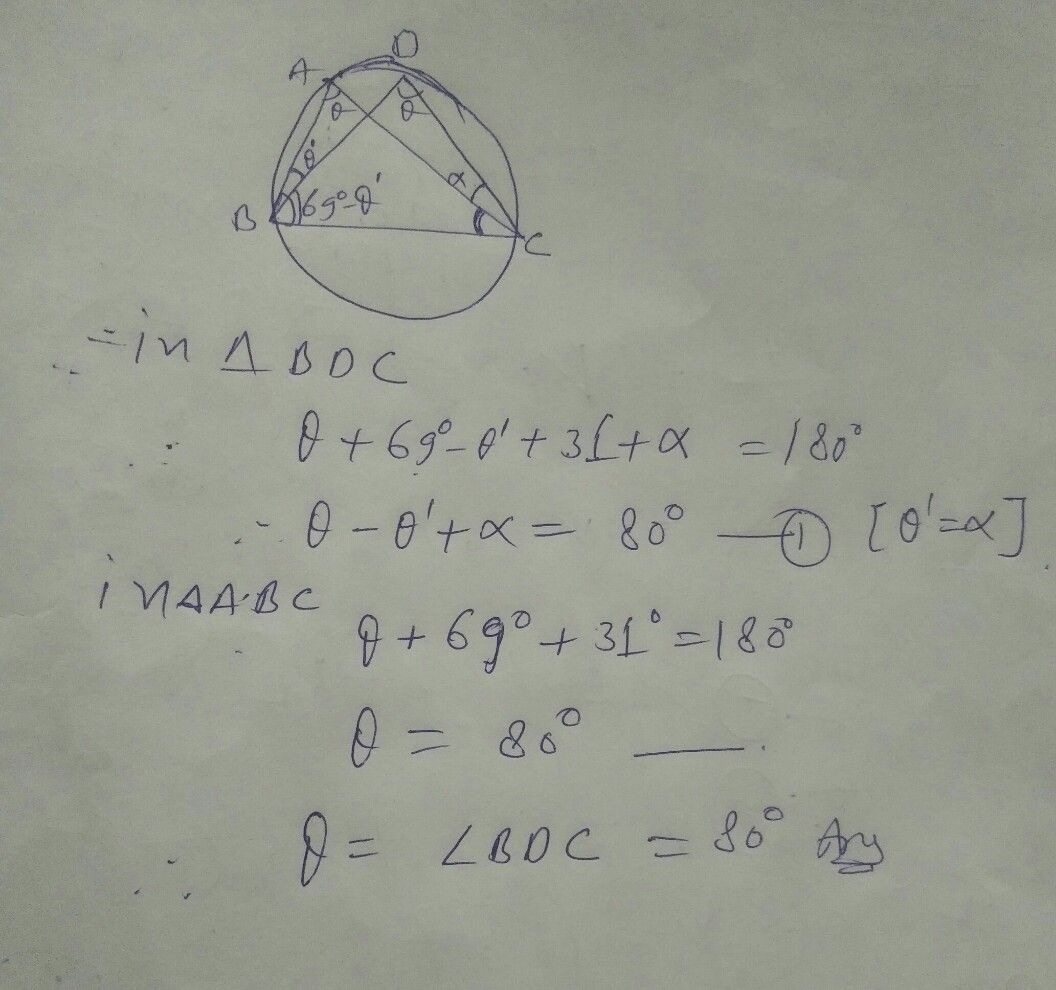Symbol
ProblemPig. $101$ In Fig. $105$ $\leq A$ $c=69.<AC10=31^{5}$ find $2$ $1$ $2$ $0$
10th-13th grade
Other
SolutionQanda teacher - tejeswar
Wait i will give yr answer